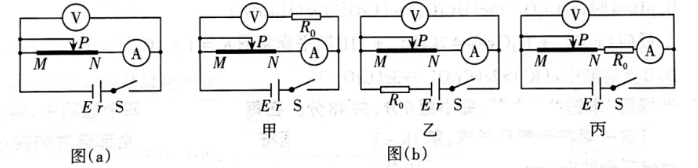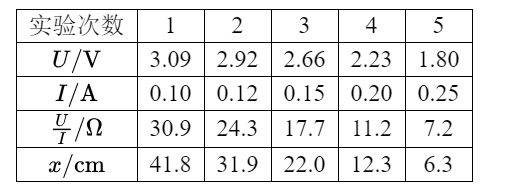(1) 图 (a) 中, $E$ 是无人机的电池, $M N$ 是他们从“热得快” 中拆下的一段粗细均匀的电热 丝。选择适当的电表量程后, 他们发现大范围移动滑片 $P$, 电压表的示数变化却不明显。 为解决这一问题, 他们在讨论后将 $R_0=8.40 \Omega$ 的定值电阻接人电路中, 那么, 选择图 (b) 中 的电路 （　　） (选填“甲”“乙”或“丙”) 可解决这一问题;(2)他们用改进后的电路进行了实验, 同时记录电压表的示数 $U$ 、电流表的示数 $I$ 及对 应的 $P N$ 的长度 $x$, 部分实验数据如下:(3) 根据表格中的 $U 、 I$ 数据, 求出该无人机电池的电动势 $E=$ （　　） $V$, 内阻 $r=$ （　　）; (结果均保留 2 位小数)
(4)根据表格中的实验数据, 在图 (c) 中补全数据点绘出 $M N$ 的 $\frac{U}{I}-x$ 图像;
(5) 已知 $M N$ 横截面积为 $3 \times 10^{-8} \mathrm{~m}^2$, 可求得 $M N$ 材料的电阻率 $\rho=$ (结果保留 1 位 有效数字)；
(6)测量 $M N$ 的电阻率时, 电流表内阻对电阻率的测量
（选填“有”或“没有”)影响。